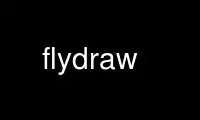# flydraw - Online in the CloudThis is the command flydraw that can be run in the OnWorks free hosting provider using one of our multiple free online workstations such as Ubuntu Online, Fedora Online, Windows online emulator or MAC OS online emulator

### PROGRAM:

#### NAME

flydraw - an inline drawing tool

flydraw

#### DESCRIPTION

flydraw is an inline drawing tool, which uses libgd to output PNG, JPG, GIF files. It
parse its standard input for drawing commands. Each command takes one line.

DRAWING COMMANDS
In the following lines, [color] may be either a color name, or 3 integers between 0 and
255, separated by commas, for the values of red,green,blue.

animate fra,del,rep
This command is only available for WIMS OEF and doc, and MUST appear on the first
line. Set up animation for fra frames, with del seconds between frames, and rep
repetitions (rep=0 means infinite repetition).

animstep n
Set up an integer which can be called in any evaluation. Used for animation. Direct
use of this command must be avoided under WIMS.

arc x,y,w,h,a1,a2,[color]
Arc segment of an ellipse of width w and hight h centered at (x,y), from angle a1
to angle a2.

arrow x1,y1,x2,y2,l,[color]
Arrow (x1,y1)--->(x2,y2), where l is the length (in pixels) of arrowhead.

circle x,y,d,[color]
Circle of center (x,y) and diameter d (in pixels).

comment
Does nothing.

copy x,y,x1,y1,x2,y2,[filename]
(Synonym: insert) Insert the region from (x1,y1) to (x2,y2) (in pixels) of
[filename] to (x,y). If x1=y1=x2=y2=-1, the whole [filename] is copied.

copyresized x1,y1,x2,y2,dx1,dy1,dx2,dy2,[filename]
Insert the region from (x1,y1) to (x2,y2) (in pixels) of [filename], possibly
resized, to the region of (dx1,dy1) to (dx2,dy2). If x1=y1=x2=y2=-1, the whole
[filename] is copied and resized.

darrow x1,y1,x2,y2,l,[color]
(Synonym: dasharrow dashedarrow) Dashed arrow (x1,y1)- - ->(x2,y2), where l is the

dhline x,y,[color]
(Synonym: dashedhorizontalline dashhorizontalline hdline horizontaldashedline)
Dashed horizontal line through (x,y).

dline x1,y1,x2,y2,[color]
(Synonym: dashedline dashline) Dashed line segment (x1,y1)---(x2,y2).

dlines [color],x1,y1,x2,y2,x3,y3...
(Synonym: dashedlines) dashlines n dashed line segments
(x1,y1)---(x2,y2)---(x3,y3)...

dvline x,y,[color]
(Synonym: dashedverticaline dashverticalline vdline verticaldashedline) Dashed
vertical line through (x,y).

ellipse x,y,w,h,[color]
Ellipse with center (x,y), width w and height h.

fcircle x,y,d,[color]
(Synonym: ball disk filledcircle) Filled circle of center (x,y) and diameter d (in
pixels).

fellipse x,y,w,h,[color]
(Synonym: filledellipse) Filled ellipse with center (x,y), width w and height h.

fill x,y,[color]
(Synonym: flood floodfill) Flood fill the region containing (x,y) with the same
original color, by color.

filltoborder x,y,[color1],[color2]
Flood fill by color2 the region containing (x,y) and bounded by color1.

fpoly [color],x1,y1,x2,y2,x3,y3...
(Synonym: filledpoly filledpolygon fpolygon) Filled polygon
(x1,y1)-(x2,y2)-(x3,y3)...

frect x1,y1,x2,y2,[color]
(Synonym: filledrect fillecrectangle frectangle) Filled rectangle with corners
(x1,y1) and (x2,y2).

fsquare x,y,s,[color]
(Synonym: filledsquare) Filled square with sides s (in pixels) and first corner at
(x,y).

ftriangle x1,y1,x2,y2,x3,y3,[color]
(Synonym: filledtriangle) Filled triangle with vertices (x1,y1),(x2,y2),(x3,y3).

hline x,y,[color]
(Synonym: horizontalline) Horizontal line through (x,y).

interlace
Set interlaced image

killbrush
Turns off brush selection for line drawing.

killtile
Turns off tile selection for filling.

lattice x0,y0,x1,y1,x2,y2,n1,n2,[color]
A lattice of n1xn2 points starting with (x0,y0), with n1 rows in direction of
(x1,y1) and n2 rows in direction of (x2,y2).

line x1,y1,x2,y2,[color]
(Synonym: seg segment) Line segment (x1,y1)---(x2,y2).

lines [color],x1,y1,x2,y2,x3,y3...
n line segments (x1,y1)---(x2,y2)---(x3,y3)...

linewidth w
Set line width to w (in pixels) for line drawing.

new x,y
Set a new image of size x,y.

output [filename]
Output the current image to [filename].

parallel x1,y1,x2,y2,xv,yv,n,[color]
n parallel lines starting from (x1,y1)---(x2,y2), with displacement (xv,yv).

pixels [color],x1,y1,x2,y2,...
Points (all of diameter 1) at (x1,y1), (x2,y2), ...

plot [color],[formula]
(Synonym: curve) Plot a curve according to [formula] which can be either an
explicit function of x, or a pair of parametric functions in t.

plotjump j
Plotted curve willjump is two consecutive points have distance more than j pixels.
Useful to avoid plotting discontinuous functions as continuous. Default value: 200.

plotstep n
(Synonym: plotsteps tstep tsteps) Set the number of point computations in curve
plot. Defaults to 100.

point x,y,[color]
A (fat) point at (x,y), whose diameter is equal to linewidth. points
[color],x1,y1,x2,y2,... (Fat) points at (x1,y1), (x2,y2), ..., whose diameters are
equal to linewidth.

poly [color],x1,y1,x2,y2,x3,y3...
(Synonym: polygon) Polygon (x1,y1)-(x2,y2)-(x3,y3)...

range x1,x2,y1,y2
Set the drawing range to [x1,x2] horizontally and [y1,y2] vertically. Note that by
default, horizontal range is [0,xsize-1] and vertical range is [ysize-1,0].

rays [color],x0,y0,x1,y1,x2,y2...
Line segments (x0,y0)---(x1,y1), (x0,y0)---(x2,y2), ...

rect x1,y1,x2,y2,[color]
(Synonym: rectangle) Rectangle with corners (x1,y1) and (x2,y2).

setbrush [filename]
Use the image [filename] as a brush for all line draws.

setpixel x,y,[color]
A point (of diameter 1 pixel) at (x,y).

setstyle [color1],[color2],...
Set the line style to color1,color2,...

settile [filename]
Use the image [filename] as a tile for all filling commands.

size x,y
Set the image size to x pixels horizontally and y pixels vertically.

square x,y,s,[color]
Square with sides s (in pixels) and first corner at (x,y).

text [color],x,y,[font],[string]
(Synonym: print string write) Write the string at (x,y), with
font=small,medium,large or giant.

textup [color],x,y,[font],[string]
(Synonym: stringup writeup) Write upwards the string at (x,y), with
font=small,medium,large or giant.

trange t1,t2
(Synonym: ranget) Set the t range to [t1,t2] for parametric curve plotting.
Defaults to [0,1].

transparent [color]
Makes [color] a transparent color.

triangle x1,y1,x2,y2,x3,y3,[color]
Triangle with vertices (x1,y1),(x2,y2),(x3,y3).

vline x,y,[color]
(Synonym: verticalline) Vertical line through (x,y).

xrange x1,x2
(Synonym: rangex) Set the horizontal drawing range to [x1,x2]. Defaults to
[0,xsize-1].

yrange y1,y2
(Synonym: rangey) Set the horizontal drawing range to [y1,y2]. Defaults to
[ysize-1,0].

#### AUTHORS

Flydraw was completely rewritten to replace a public domain program called Fly by XIAO
Gang from the University of Nice (France) in April 2000.

Use flydraw online using onworks.net services

Free Servers & Workstations